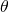# Gaussian Optics

is another term for paraxial optics.

Carl Friedrich Gauss (1755-1855) laid in 1840 the foundations for this subject. In his work “Dioptrische Untersuchungen” Gauss showed, that the behaviour of any lens system can be determinedform the knowledge of it’s six cardinal points (also called Gauss-Points), namely two focal points, two nodal points (of unit angular magnification)  and two principal points (of unit linear magnification) .

Included in the paper were recipes for experimentally determining the positions of these points and iterative methods for calculating them in terms of the surface curvatures, separations and refractive indices of the lens system.

He explicitely mentioned  two equations where ray height r and ray angleof an input and output ray are linked, as used in the the ABCD-Matrix.But Matrix notation wasn’t known at that time. So instead he used an algorithm he learnt from Leonard Euler. It was a shorthand form of continuous fraction, now known as Gaussian Brackets.

It’s only allowed to use ABCD Matrices in the paraxial range, withThe anglesandare measured in radians!

For a table of ray transfer matrixes for simple optical components see ABCD matrix